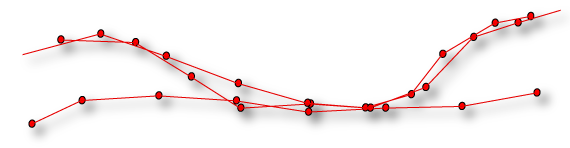# ArcMET Manual

 TOOLSET TOOL DESCRIPTION Filter Filter Apply a movement, temporal, day-hour and/or spatial filter to movement trajectory MovWin Dates Create a moving window dates file for use with ArcMET tools Trajectory Resample Re-sample/downsample a movement trajectory Path Create a trajectory path from a movement trajectory Path Statistics Calculate statistics (e.g., mean, min, max, etc.) for trajectory path properties (e.g., distances, speeds, headings) Path Metrics Calculate trajectory path metrics (e.g., Tortuosity, displacement) Temporal Metrics Calculate statistics and metrics about the time-spans between recorded fixes Velocity Grid Create landscape level summary of trajectory path segments (e.g., speeds, headings, directional similarity) Conspecific Proximity Calculate conspecific inter-distance & headings for temporally overlapping trajectories Range MCP Calculate a Minimum Convex Polygon Range aLoCoH Calculate a ’adaptive’ Local Convex Hull Range UD KDE Calculate a Kernel Density Estimator UD LTD Calculate a Linear Time-Density UD BBMM Calculate a Brownian Bridge Movement Model UD TGDE Calculate a Time-Geographic Density Estimator UD ETD Calculate a Elliptical Time-Density UD Percent Contours Calculate UD percentile contours Covariate Regional Raster Stats Calculate raster statistics within polygon regions with temporal selection option Utilities Spatial Integrator Calculate the sum or density of point, line, polygon features within raster or polygon-grid pixels Polygon Grid Generate a polygon grid feature class Map Extent Polygon Generate a rectangular polygon set to the current map/display extent Garmin GPX Import Import GPX data from Garmin device Temporal Selection Click a feature with time info and automatically select all features within +/- hrs